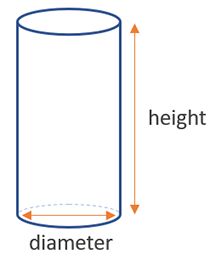# Volume of a Cylinder Calculator

Use this cylinder volume calculator to easily calculate the volume of a cylinder from its base radius and height in any metric: mm, cm, meters, km, inches, feet, yards, miles...

### Calculation results

785.398163 cm3 Cylinder volume
Share calculator:

Embed this tool:
get code

## Volume of a cylinder formula

The formula for the volume of a cylinder is height x π x (diameter / 2)2, where (diameter / 2) is the radius of the base (d = 2 x r), so another way to write it is height x π x radius2. Visual in the figure below:First, measure the diameter of the base (usually easier than measuring the radius), then measure the height of the cylinder. To do the calculation properly, you must have the two measurements in the same length units. The result from our volume of a cylinder calculator is always in cubic units, based on the input unit: in3, ft3, yd3, cm3, m3, km3, and so on.

## How to calculate the volume of a cylinder?

One can think of a cylinder as a series of circles stacked one upon another. The height of the cylinder gives us the depth of stacking, while the area of the base gives us the area of each circular slice. Multiplying the area of the slice by the depth of the stack is an easy way to conceptualize the way for calculating the volume of a cylinder. Since in practical situations it is easier to measure the diameter (of a tube, a round steel bar, a cable, etc.) than it is to measure the radius, and on most technical schemes it is the diameter which is given, our cylinder volume calculator accepts the diameter as an input. If you have the radius instead, just multiply it by two.

Using the formula and doing the calculations by hand can be difficult due to the value of the π constant: ~3.14159, which can be hard to work with, so a volume of a cylinder calculator significantly simplifies the task.

## Example: find the volume of a cylinder

Applying the volume formulas is easy provided the cylinder height is known and one of the following is also given: the radius, the diameter, or the area of the base. For example, if the height and area are given to be 5 feet and 20 square feet, the volume is just a multiplication of the two: 5 x 20 = 100 cubic feet.

If the radius is given, using the second equation above can give us the cylinder volume with a few additional steps. For example, the height is 10 inches and the radius is 2 inches. First, we find the area by 3.14159 x 22 = 3.14159 x 4 = 12.574, then multiply that by 10 to get 125.74 cubic inches of volume. Using a higher level of precision for π results in more accurate results, e.g. our calculator computes the volume of this cylinder as 125.6637 cu in.

## Practical applications

The cylinder is one of the most widely used body shapes in engineering and architecture: from tunnels, covered walkways to tubes, cables, round bars, the cylinders and pistons in your car's engine - cylinders are everywhere. Calculating cylinder volume is useful when you want to know its displacement, or how much liquid or gas you need to fill it, e.g. how much water you need to fill your jacuzzi. Cylindrical aquariums are also fairly common, so are cylindrical artificial lakes, fountains, gas containers / tanks, etc.

#### Cite this calculator & page

If you'd like to cite this online calculator resource and information as provided on the page, you can use the following citation:
Georgiev G.Z., "Volume of a Cylinder Calculator", [online] Available at: https://www.gigacalculator.com/calculators/volume-of-cylinder-calculator.php URL [Accessed Date: 27 Mar, 2023].